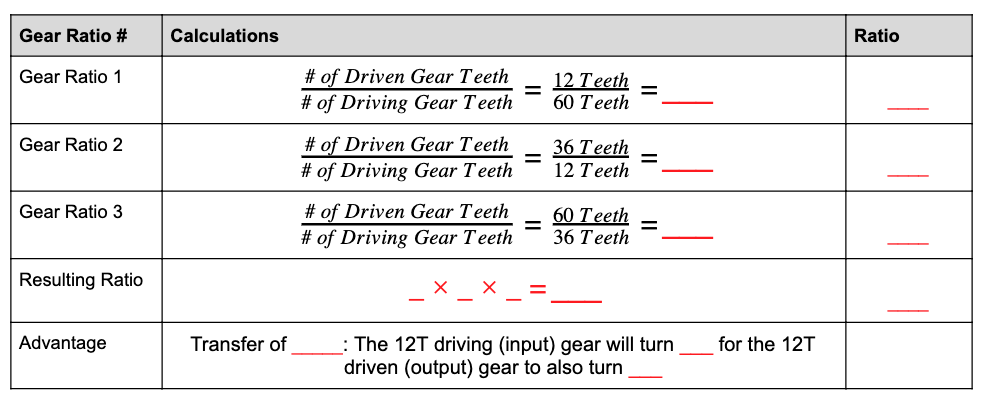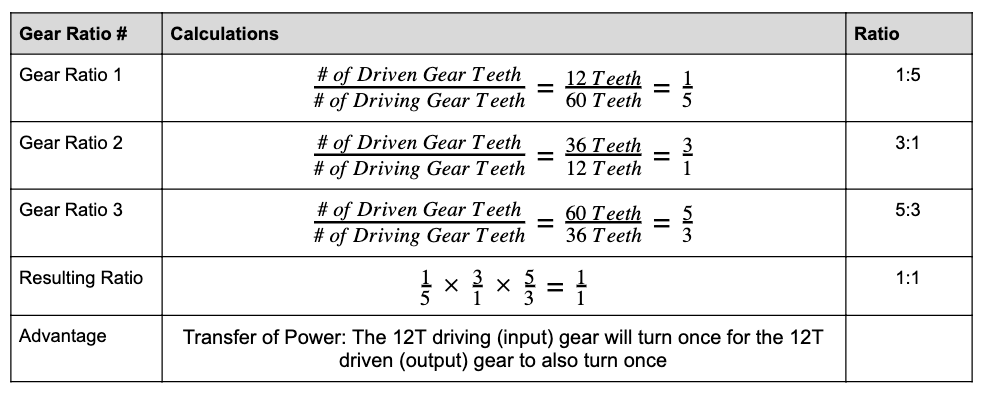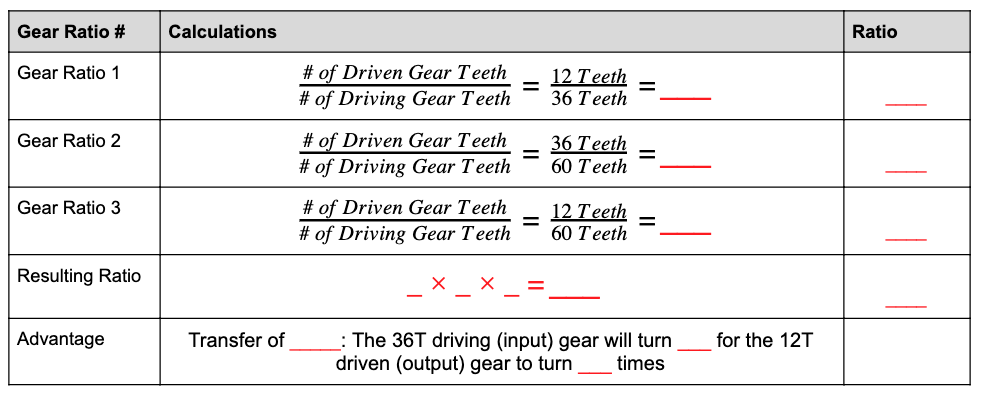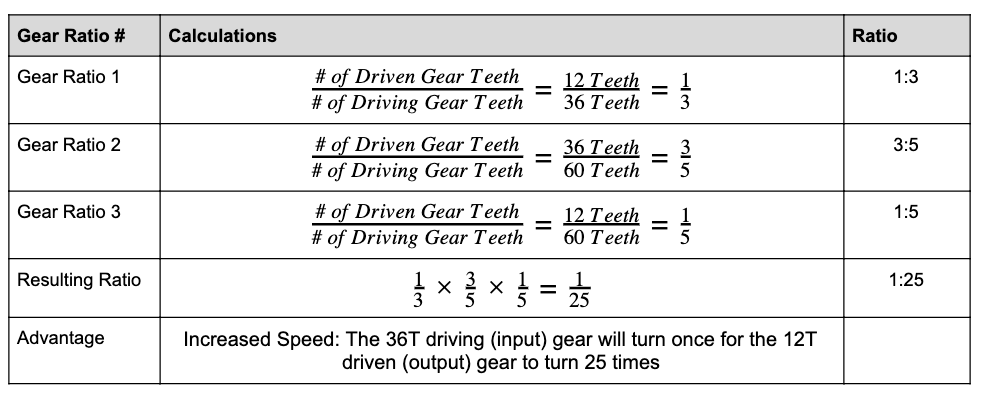STEMLabs IQ
Lab 12 - M.A.D. Box

Teacher PortalTeacher Tips - Vocabulary

Numerator: the top part of a fraction.
Denominator: the bottom part of the fraction.

Now that you have calculated a compound gear ratio from two gear ratios, we will now calculate a compound gear ratio from three gear ratios!

You will work in groups of four to calculate gear ratios and determine the resulting mechanical advantage.

### Calculation 2Teacher Tips - Fraction Simplification

Students often struggle when simplifying fractions. 12/60 and 36/12 will simplify evenly with a 1 in either the numerator or denominator.

However, 60/36 does not simplify evenly with a 1 in either the numerator or denominator. Ensure that students are aware that the most that this fraction can simplify down to is 5/3 by diving each part of the fraction by 12.

Fill in the missing calculations from the Gear Ratio table. Keep in mind, each person should be calculating according to their role.• Role 1: Calculate the Gear Ratio 1 row of the above table. Show all work in your engineering notebook.

• Role 2: Calculate the Gear Ratio 2 row of the above table. Show all work in your engineering notebook.

• Role 3: Calculate the Gear Ratio 3 row of the above table. Show all work in your engineering notebook.

• Role 4: Calculate the Resulting Ratio row of the above table. Check the calculations from Gear Ratio 1, 2, and 3 before calculating the final compound gear ratio. Show all work in your engineering notebook.

• All Roles: Calculate the Advantage row of the above table. Show all work in your engineering notebook.Teacher Toolbox - Solution

Provided is the following solution table for Calculation 2. The mechanical advantage for Calculation 2 is a 1:1 ratio, thus resulting in a Transfer of Power.Teacher Toolbox - Stop and Discuss

A 1:1 ratio could have also been achieved using only two gears of the same size. Initiate a discussion by asking your students the following:

• When would a 1:1 gear ratio be helpful on a robot?

• Were all three gear ratios necessary for achieving a compound gear ratio of 1:1? If not, can you provide an example of one that uses less gears?

• Can you explain in a sentence what a 1:1 gear ratio is in regards to how many times each girl will turn? (Hint: The __T driving (input) gear will turn once for the __T driven (output) gear to also turn once).

### Calculation 3

Fill in the missing calculations from the Gear Ratio table. Keep in mind, each person should be calculating according to their role.• Role 1: Calculate the Gear Ratio 1 row of the above table. Show all work in your engineering notebook.

• Role 2: Calculate the Gear Ratio 2 row of the above table. Show all work in your engineering notebook.

• Role 3: Calculate the Gear Ratio 3 row of the above table. Show all work in your engineering notebook.

• Role 4: Calculate the Resulting Ratio row of the above table. Check the calculations from Gear Ratio 1, 2, and 3 before calculating the final compound gear ratio. Show all work in your engineering notebook.

• All Roles: Calculate the Advantage row of the above table. Show all work in your engineering notebook.Teacher Toolbox - Solution

Provided is the following solution table for Calculation 3. The mechanical advantage for Calculation 3 is a 1:25 ratio, thus resulting in Increased Speed.Teacher Toolbox - Mechanical Advantage

Now that students have calculated gear ratios that result in all three types of mechanical advantage (Transfer of Power, Increased Speed, and Increased Torque) encourage students to reflect on their calculations from their engineering notebooks. Ask students to identify any patterns that they noticed. Ask the students the following questions:

Q: What patterns did you notice when calculating a ratio that resulted in a Transfer of Power?
A: Answers may vary, but students should note that the compound gear ratio will simplify down to a fraction of 1/1.

Q: What patterns did you notice when calculating a ratio that resulted in Increased Speed?
A: Answers may vary, but students should note that the first part of the ratio that is represented by the numerator of the fraction will be a smaller number compared to the second part of the ratio or the denominator of the fraction. In other words, the driving input gear will turn less times compared to the driven output gear.

Q: What patterns did you notice when calculating a ratio that resulted in Increased Torque?
A: Answers may vary, but students should note that the second part of the ratio that is represented by the denominator of the fraction will be a smaller number compared to the first part of the ratio or the numerator of the fraction. In other words, the driving input gear will turn more times compared to the driven output gear.

Q: What is the overall relationship between Speed and Torque?
A: As speed increases, torque decreases and vice versa. This can be seen by their respective gear ratios.